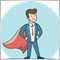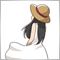I dont Found SomeThing In this Indicator ? Important - page 2569

What Mladen wrote is correct.

Did you insert it in the correct position?

For example, it should be put in the next line of "# property indicator_plots 3" in the above program.

yes i put in this position

and when attach indicator show me this option open And thats good

but problem here

when i connect this indicator after above change with my projects EA , when indicator attach auto when my ea work i found this option become close ( I dont how or why ?)

So , i think we must change in the code direct somethings not add new code :)

thank you475

Return the header to the usual one and then change the "price" in the program to "open".

//+------------------------------------------------------------------+
//| Custom indicator iteration function                              |
//+------------------------------------------------------------------+
int OnCalculate(
const int rates_total,
const int prev_calculated,
const datetime &time[],
const double &open[],
const double &high[],
const double &low[],
const double &close[],
const long &tick_volume[],
const long &volume[],
)
{
//---
int start_bar;
bool up;
static bool _up;
//---
if ( prev_calculated > rates_total || prev_calculated <= 0 ) {
for ( start_bar = 0; MathAbs(open-open[start_bar]) < box_size; start_bar++ ) {}
if ( open[start_bar] > open ) {
UpperBuffer[start_bar] = open[start_bar];
LowerBuffer[start_bar] = open;
BoxesBuffer[start_bar] = 1.0;
_up = true;
} else {
UpperBuffer[start_bar] = open;
LowerBuffer[start_bar] = open[start_bar];
BoxesBuffer[start_bar] = -1.0;
_up = false;
}
start_bar += 1;
for ( int plot = 0; plot < 3; plot++ ) {
PlotIndexSetInteger(0, PLOT_DRAW_BEGIN, start_bar);
}
} else {
start_bar = prev_calculated - 1;
}
//---
up = _up;
//---
for ( int bar = start_bar; bar < rates_total; bar++ ) {
double prev_up = UpperBuffer[bar-1];
double prev_dn = LowerBuffer[bar-1];
double prev_boxes = BoxesBuffer[bar-1];

if ( open[bar] >= prev_up + box_size ) {
UpperBuffer[bar] = open[bar];
LowerBuffer[bar] = prev_up;
if ( up ) {
BoxesBuffer[bar] = prev_boxes + 1;
} else {
up = true;
BoxesBuffer[bar] = 1.0;
}
} else if ( open[bar] <= prev_dn - box_size ) {
UpperBuffer[bar] = prev_dn;
LowerBuffer[bar] = open[bar];
if ( up ) {
up = false;
BoxesBuffer[bar] = -1;
} else {
BoxesBuffer[bar] = prev_boxes - 1;
}
} else {
UpperBuffer[bar] = prev_up;
LowerBuffer[bar] = prev_dn;
BoxesBuffer[bar] = prev_boxes;
}

if ( bar < rates_total - 1 ) {
_up = up;
}
}
//--- return value of prev_calculated for next call
return(rates_total);
}
//+------------------------------------------------------------------+569